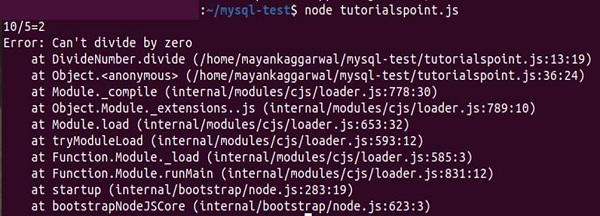# NodeJS - Exception Handling in eventful Code

An exception is a type of an event, that occurs while executing or running a program that stops the normal flow of the program and returns to the system. When an exception occurs the method creates an object and gives it to the runtime system. This creating an exception and giving it to the runtime system is known as throwing an Exception.

We need to handle these Exceptions to handle any use case and prevent the system from crashing or performing an unprecedented set of instructions. If we donot handle or throw exceptions, the program may perform strangely.

## Exception handling in Synchronous Program

Here, we will learn how to handle exceptions in a eventful flow of program. In an eventful flow of program, an error can occur anywhere. Therefore, instead of throwing the error, you need to fire the error event instead.

## Example

// Definite our Divide Number Event
var events = require("events")
var DivideNumber = function(){
events.EventEmitter.call(this)
}
require('util').inherits(DivideNumber, events.EventEmitter)

DivideNumber.prototype.divide = function(x, y){
// if error condition occurs ?
if ( y === 0 ) {
// "throw" the error safely by emitting it
var err = new Error("Can't divide by zero")
this.emit("error", err)
} else {
// If No error occurrs, return the result
this.emit("Number divided", x, y, x/y)
}

// Return the event chain
return this;
}

// Create our divider and listen for errors
var divideNo = new DivideNumber()
divideNo.on('error', function(err){
// handle the error safely
console.log(err)
})
divideNo.on('Number divided', function(x, y, result){
console.log(x+"/"+y+"="+result)
})

// Dividing the number
divideNo.divide(10, 5).divide(10, 0)

## Output